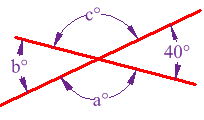# if two straight lines intersect each other prove that the ray opposite to the bisector of one of the angles thus formed bisects the vertically opposite anglesplz answer this immediately , its most urgent

Hi!Cheers!

• 45In this example, a° and b° are vertical angles.

The interesting thing here is that vertically opposite angles are equal:

• 6Because b° is vertically opposite 40°, it must also be 40°

A full circle is 360°, so that leaves 360° - 2×40° = 280°

Angles a° and c° are also vertically opposite, so must also be equal, which means they are 140° each.

Answer: a = 140°, b = 40° and c = 140°.

• -10

My writing mistake in the first one , sorry :(

• -9
What are you looking for?